# Expedition ML4SEC Part – 1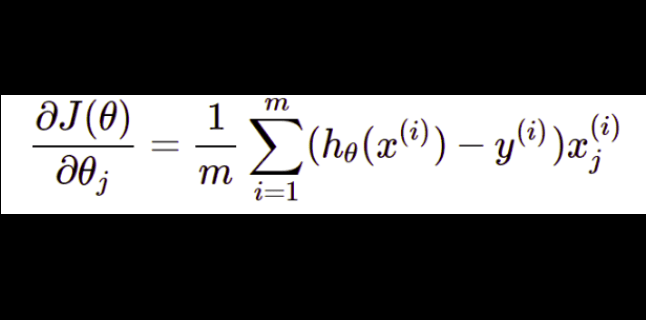Machine learning is becoming a very ubiquitous technology. It is used to solve problems that are difficult or impossible to be solved by defining explicit algorithms. For example, problems like classifying malicious HTTP requests from non-malicious HTTP requests, determining intrusive actions from logs, etc. Machine learning algorithms do a very good job in solving such problems. Hence these algorithms are being used by well known defensive security solutions like firewalls, antiviruses, honeypots, IDS/IPS systems, etc.

There is no doubt that state-of-the-art systems can be built using machine learning algorithms but at the same time these algorithm poses serious security flaws. An attacker can take advantage of these flaws by creating adversarial inputs resulting in misbehaviour of Machine Learning systems. In this series we will explore these flaws. But to understand about the vulnerabilities we first need to understand how machine learning models work. Hence, I will dedicate this post to understand the basics of machine learning and finish with building a basic machine learning model.

### Introduction:

Training a machine learning model means increasing the performance of that model in a particular task. Model “Learns” from a dataset. Based on this learning process, machine learning algorithms are classified into following major types.

Supervised learning: In supervised learning, we feed the algorithm with features and labels. Consider a problem of classifying network packets into malicious or non malicious. Here features could be the attributes of packet such as source IP, destination IP, port, protocol, payload length, flags,etc. And the labels could be 0 or 1 based based on whether the packet is malicious or not. Classification algorithms like Neural Nets and SVM.

Unsupervised learning: This type of learning is used when we do not have a labeled samples. Algorithms learns to differentiate the samples based on the features. Suppose we have a huge set of images of 2 persons and want to classify them. Then we feed these images to an unsupervised algorithm. The algorithm will then create two or more clusters of these images(based on features), which can be labelled as person A and person B. Hence these algorithms are sometimes called as “clustering” algorithms.

Semi-supervised learning: Semi-supervised learning is used when we have a mixture of labeled and unlabeled data in dataset.

Following image is designed by Scikit-learn provides a good visualisation of different machine learning techniques and when to use them.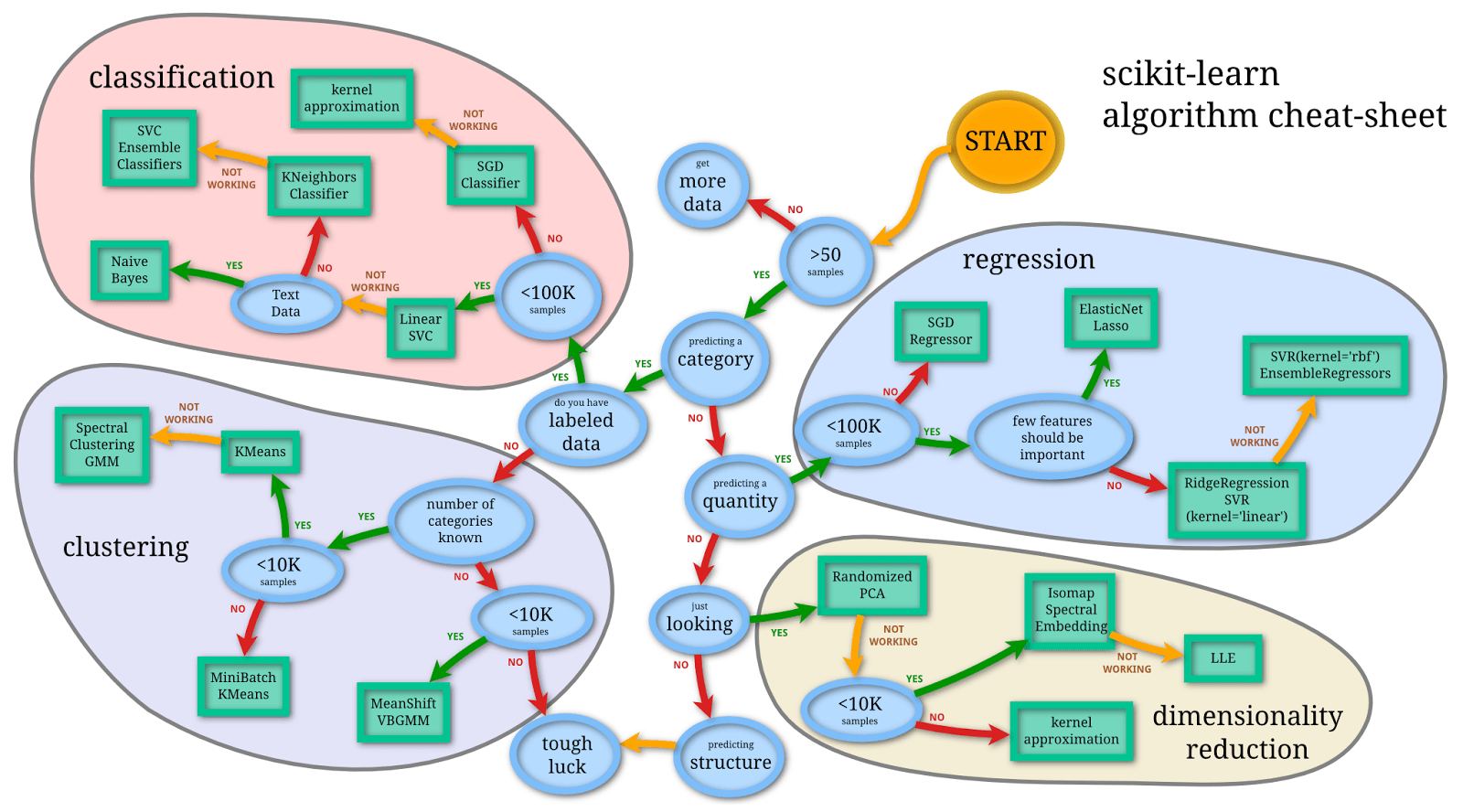### Understanding the Hello World!

Let’s start with the ‘Hello World!’ of machine learning. We will try to solve a classic problem to classify the Iris flower into its types. We will be using scikit-learn to complete this task. Scikit-learn is an open source machine learning library for the Python programming language and it is perfect for beginners.

Dataset description: you can download the dataset from here. However scikit-learn provides this dataset in the library itself. Dataset contains three classes (Iris-sentosa, Iris-versicolor and Iris-virginica) of iris flower shown below.You can clearly see that the sizes of sepals and petals of flower varies with the class. Hence based on these feature we are going to classify the flowers. You can see that there are five columns in the dataset. Each representing following attribute.Column Information:
1. sepal length in cm
2. sepal width in cm
3. petal length in cm
4. petal width in cm
5. class: Iris Setosa (0), Iris Versicolour (1), Iris Virginica (2)

The final aim is to build a model which learns from above four features and predicts the type of Iris flower when features are provided as input.

In this post we will be trying out two well known machine learning techniques for our classification task. One is Logistic Regression and other is Artificial Neural networks. I will first give a brief insight of how these algorithms work. So get ready for some Maths!!

Although to implement these methods you don’t need to know about underlying mathematics, but where’s the fun in that ;-). Feel free to skip this part and jump to implementation.

#### Mathemagic: Logistic regression

Logistic regression can be used for both binary and multiclass classification. Logistic regression tries to build a decision boundary around the data points which can be used for classification. In layman terms we are plotting lines which can separate one set of points from others based on features, as shown in figure below.But how can we tell the computer to do that? So, let me introduce you the logistic function represented by following equation.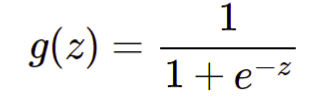Logitic function is also known as sigmoid function. You can see that the sigmoid function will always output values between 0 and 1. Hence we can use it in our hypothesis function shown below to classify data into class 0 and 1.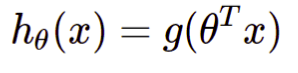Following equation represents the cost function.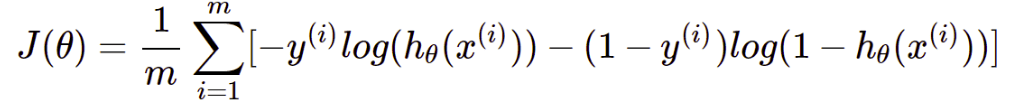We are supposed to reduce the cost using the magic of differential calculus. Which is shown in equation below. I will not go in much depth about how these equations work. It will need a dedicate post to explain the beauty of these equations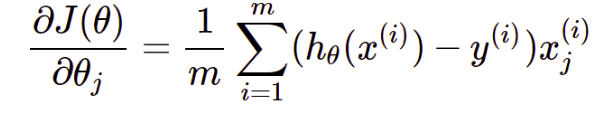We can now classify data into two classes, but in our example there are three classes! It’s time to pull the oldest trick in the book, i.e. to use one-vs-all classification. Meaning, we will draw a line which separates Iris-Sentosa from everything which is not Iris-Sentosa. And repeat this step for all other classes of Iris.

#### Mathemagic: Artificial neural nets

A long time (~70 years) ago, papers were written explaining a mathematical model which simulates the behaviour of neurons in brain. It unveiled the process of learning. Later, computers became powerful enough to run those models. Neural nets became very popular and are being used extensively in machine learning.

Neuron is a basic functional unit of neural net and our brain. Image below mathematically describes a single neuron.It has a set of inputs X and weights W. Sum of the products of X and  W is then passed to an activation function f() and the result is passed to other neurons for further processing.

A neural net is a network of such neurons with an input layer and an output layer. There could be multiple hidden layers with varying number of neurons in them.In our case we will be having 4 neurons in input layer, as we have four features and 3 neurons in output layer representing the class of input feature.

To train a neural network, we need to adjust the weights of neural net in such a way that it can accurately predict the class when provided with input features. To achieve this there are multiple optimisation algorithms and one of them is backpropagation. Check out this link to get thorough idea of backpropagation. In simple words, it will  first initialise the weights W randomly and re-adjusts the weights based on the difference between generated prediction and actual class values. This step continues for n number of iterations.

### Hello World! – Implementation

Now we have the knowledge of algorithms and the required dataset. We can use it for implementation. Scikit-learn provides Linear Regression and Neural Nets as predefined functions. It also provides the required dataset. We can start working on the dataset just like we use numpy arrays.

```from sklearn import datasets
from sklearn.linear_model import LogisticRegression
from sklearn.metrics import accuracy_score
from sklearn.neural_network import MLPClassifier
from sklearn.model_selection import train_test_split
import pickle
X_train, X_test, y_train, y_test = train_test_split(dataset.data,
dataset.target, test_size=0.33)
```

I have used the train_test_split() function to split the data set into training and testing data. X_train and y_train are training features and targets/classes respectively. X_test and y_test will be used for testing. test_size specifies the fraction of dataset that will be used for testing.

```model_lr = LogisticRegression()
model_nn = MLPClassifier()
```

Using LogisticRegression() and MLPClassifier() I have initialised the models with default parameters. MLP is a type of neural network which stands for Multi Layer Perceptron. To explore more about the functions you can visit the documentation. Tuning the parameters of these models can increase their accuracy.

```for model in [model_lr, model_nn]:
model.fit(X_train, y_train)
# predict
predicted = model.predict(X_test)
# calculate accuracy
accuracy = accuracy_score(y_test, predicted)
print(str(model.__class__)+": Accuracy: %.2f%%" % (accuracy * 100.0))
```

In above code snippet I am using the fit() function to train the models with our training data set. I have calculated the accuracy to compare the models. Different metrics can be used for comparision, but we will stick to accuracy. Notice that while calculating accuracy I am using the testing dataset. Following output is obtained after running the code. It shows that the accuracy of MLP is more than that of Logistic regression. 96% is not the best accuracy that we can get, there is still scope to fine tune the parameters of model.

```<class 'sklearn.linear_model.logistic.LogisticRegression'>: Accuracy: 94.00%
<class 'sklearn.neural_network.multilayer_perceptron.MLPClassifier'>: Accuracy: 96.00%
```

We can use serialisation to save the trained models on disk and use them later. I am using pickle to save the model as shown below. It will be saved as a binary file with name pickled_ml.

```with open('pickled_ml','wb') as f:
pickle.dump(model_nn, f)
```

You can load the model from disk and use it just like a python object. Loaded model can be used for prediction. I have given an input which resembles to IrisVersicolour and the model generated correct output.

```with open('pickled_ml','rb') as f:

# use model for prediction
iris_type = ['Iris Setosa', 'Iris Versicolour', 'Iris Virginica']
res = loded_model.predict([[5.6,2.9,4.4,1.2]])
print iris_type[res]
```

output:

```Iris Versicolour
```

Complete code is given below. Feel free to try out different datasets and algorithms. In case you are wondering, here you can find security related datasets.

```from sklearn import datasets
from sklearn.linear_model import LogisticRegression
from sklearn.metrics import accuracy_score
from sklearn.neural_network import MLPClassifier
from sklearn.model_selection import train_test_split
import pickle
X_train, X_test, y_train, y_test = train_test_split(dataset.data,
dataset.target, test_size=0.33)
# fit a logistic regression model to the data
model_lr = LogisticRegression()
model_nn = MLPClassifier()
for model in [model_lr, model_nn]:
model.fit(X_train, y_train)
# predict
predicted = model.predict(X_test)
# calculate accuracy
accuracy = accuracy_score(y_test, predicted)
print(str(model.__class__)+": Accuracy: %.2f%%" % (accuracy * 100.0))

with open('pickled_ml','wb') as f:
pickle.dump(model_nn, f)
with open('pickled_ml','rb') as f:

# use model for prediction
iris_type = ['Iris Setosa', 'Iris Versicolour', 'Iris Virginica']
res = loded_model.predict([[5.6,2.9,4.4,1.2]])
print iris_type[res]```

That’s all for this post. I hope you had a great time while learning the process of learning.

In upcoming posts we will explore the  vulnerabilities in machine learning models and how to leverage these vulnerabilities. For example, tricking an image classifier, bypassing a firewall that uses machine learning, bypassing a malware detector, etc. So stay tuned for an epic expedition!

### References:

Fill in your details and get your copy of the datasheet in few seconds

Fill in your details and get your copy of the ebook in few seconds

Fill in your details and get your copy of sample report in few seconds

Fill in your details and get your copy of sample report in few seconds

Fill in your details and get your copy of sample report in few seconds

Fill in your details and get your copy of sample report in few seconds

Fill in your details and get your copy of sample report in few seconds

Fill in your details and get your copy of sample report in few seconds

Fill in your details and get your copy of sample report in few seconds

Fill in your details and get your copy of sample report in few seconds

Fill in your details and get your copy of sample report in few seconds

Fill in your details and get your copy of sample report in few seconds